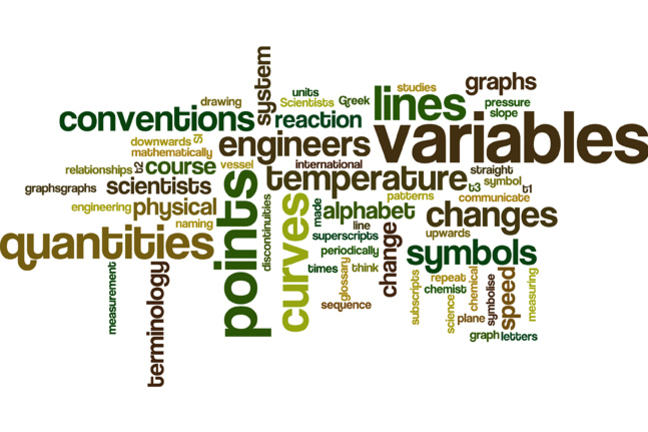1.17

Loughborough UniversitySummary of Week 1

We now summarise all that we have learned:

We started by explaining that scientists and engineers are interested in physical quantities - such as speed, pressure, temperature - and how these quantities change in response to changes in other quantities. We called these changing quantities variables. We symbolised these variables using letters of the alphabet - sometimes the Greek alphabet. We learned that not only should we choose (as far as possible) meaningful and helpful letters - so for example $T$ might be used as a symbol for temperature - but we can also attach subscripts and superscripts to symbols in order to extend our collection of symbols in a meaningful way. So, for example, a sequence of times at which a measurement is made could be labelled $t_1$, $t_2$, $t_3$ and so on.

We then discussed how scientists and engineers symbolise or represent changes in variables in response to changes in other variables. For example, a chemist might want to represent change in the speed of a chemical reaction as the temperature of the reaction vessel is increased. A very common, pictorial way of representing relationships between variables is to use graphs.

We laid the foundations for what will follow in Weeks 2 and 3 by explaining that graphs are constructed by drawing points on a plane and then joining points to produce lines and curves. Many important relationships between variables can be represented by straight line graphs. We encouraged you to think about and describe some graphs in order that you begin to think mathematically about the meaning behind them:

• whether lines sloped upwards, downwards, or not at all,
• whether patterns repeated periodically,
• whether lines had breaks in them - discontinuities,

and so on.

We provided information about measuring conventions and naming conventions. Scientists and engineers have developed terminology in order that physical quantities can be described precisely and so that they can communicate with each other and be sure that they are all talking about the same thing! We drew particular attention to the international system of units - the SI system: Le Système International d’Unités. You will gradually become accustomed to these conventions as your studies in science or engineering progress. You don’t have to learn all this now - but through exposure to it you will gain the required familiarity.

Finally, let us remind you that a glossary is provided at the end of the week’s course material and we will build this up as more terminology is introduced in the remaining weeks of the course.